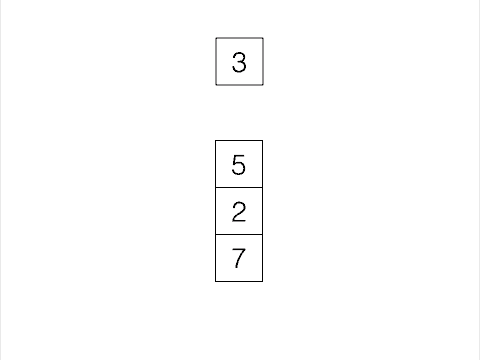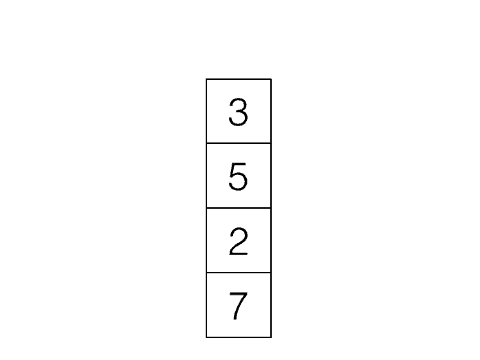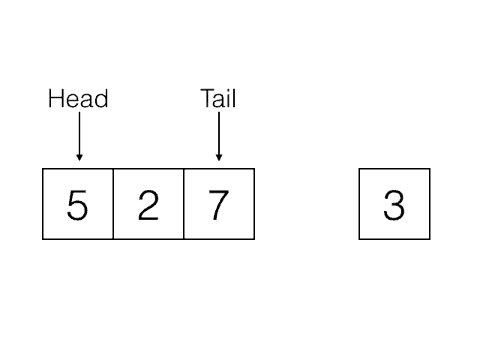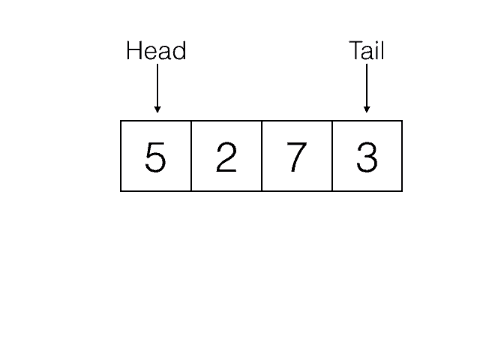# Queues and Stacks

This is part of the Algorithms with Auntie Aja series.

This is part of my series on algorithms and data structures called “Algorithms with Auntie Aja”. This series provides an introduction data structures and algorithms for folks who never learned it or have forgotten it.

### Algorithms with Auntie Aja: Stacks and Queues

I wanted to start this series with basic maze solving using breadth first search. That was one of the first applications (and algorithms) I learned in my CS courses and it has a soft spot in my heart. I realized, though, that understanding breadth-first search requires understanding queues. Likewise understanding depth-first search requires stacks. So I’ll be starting this series with queues and stacks.

### Stacks

A stack is one of the simplest data structures. It is just like a pile of playing cards or a stack of plates. When you add something to the pile you put it on top of all the things that are already there. When you take something away you remove it from the top of the stack. Computer Science uses specialized terms for things like this though. So instead of add we say push, and instead of remove we say pop. Another term you may hear with regards to stacks is LIFO which stands for Last In First Out. With a stack the last thing you added in the first thing that is removed.

##### Push##### Pop#### Implementing a stack

Here are some basic tests for what a stack should be able to do:

 ```1 2 3 4 5 6 7 8 9 10 11 12 13 14 15 16 17 18 19 20 21 22 23 24 25 26 27 28 29 30 ``` ```class TestStack < Minitest::Test def test_push_pop stack = Stack.new stack.push 3 assert_equal 3, stack.pop end def test_multi_push_pop stack = Stack.new stack.push 3 stack.push 5 assert_equal 5, stack.pop assert_equal 3, stack.pop end def test_empty stack = Stack.new assert stack.empty? stack.push 3 refute stack.empty? stack.pop assert stack.empty? end end ```

A super simple stack implementation uses Ruby’s dynamic arrays and the built in `push` and `pop` methods. You might be more familiar with `push` as `>>` or shovel (although they are slightly different).

 ```1 2 3 4 5 6 7 8 9 10 11 12 13 14 15 16 17 ``` ```class Stack def initialize @data = [] end def push a @data.push a end def pop @data.pop end def empty? @data.empty? end end ```

If you don’t want to use the built in methods here is another implementation that keeps track of the index of the last item put on the stack.

 ```1 2 3 4 5 6 7 8 9 10 11 12 13 14 15 16 17 18 19 20 21 22 ``` ```class Stack def initialize @data = [] @head = -1 end def push a @data << a @head += 1 end def pop result = @data[@head] @data.delete_at(@head) @head -= 1 result end def empty? @head == -1 end end ```

### Queues

You probably have experience with physical queues at amusement parks, concerts, or sports arenas. In the US, we usually call them lines. A queue is an ordered collection (or group) where we add things to one end (called the tail) and we remove things from the other end (called the head). Adding things to a queue is called enqueueing. Removing items from a queue is called dequeueing. This is also called FIFO for first in, first out.

##### Enqueue##### Dequeue#### Implementing a queue

Here are the tests for a basic queue implementation.

 ```1 2 3 4 5 6 7 8 9 10 11 12 13 14 15 16 17 18 19 20 21 22 ``` ```class TestQueue < Minitest::Test def setup @queue = Queue.new end def test_enqueue_one_item @queue.enqueue 3 assert_equal 3, @queue.dequeue end def test_equeue_and_dequeue @queue.enqueue 3 @queue.enqueue 5 assert_equal 3, @queue.dequeue assert_equal 5, @queue.dequeue end def test_empty? assert @queue.empty? end end ```

Like with stack the Array class has some built in methods that make implementing a queue easy. `shift` removes the first item in the array and returns it. This is dequeue. `<<` can be used to add items to the end of the queue. Ruby arrays also have `unshift` which adds an item to the front of an array. This isn’t needed to implement a queue or a stack. Here’s the basic queue implementation.

 ```1 2 3 4 5 6 7 8 9 10 11 12 13 14 15 16 17 ``` ```class Queue def initialize @data = [] end def enqueue item @data << item end def dequeue @data.shift end def empty? @data.empty? end end ```

And here’s a version that doesn’t use the built in methods. It is very similar to the stack implementation above.

 ```1 2 3 4 5 6 7 8 9 10 11 12 13 14 15 16 17 18 19 20 21 22 ``` ```class Queue def initialize @data = [] @tail = -1 end def enqueue item @data << item @tail += 1 end def dequeue result = @data @data.delete_at(0) @tail -= 1 result end def empty? @tail == -1 end end ```

### Conclusion and Bonus

These two data structures are the heart of Breadth-First Search and Depth-First Search. In turn those algorithms can be used to solve mazes, build minesweeper, and solve a large number of path-finding problems. The next post will explain those algorithms and show how they use these data structures.

If you want to play around with data structures more, try implementing a stack or a queue using a linked list instead of an array. If you get stuck try drawing out your linked list on paper and walking through a simple test case. It is what I do and it usually helps.